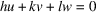International
Tables for
Crystallography
Volume C
Mathematical, physical and chemical tables
Edited by E. Prince

International Tables for Crystallography (2006). Vol. C, ch. 1.3, p. 12

Section 1.3.3. Implication of twinning in reciprocal space

E. Kocha

aInstitut für Mineralogie, Petrologie und Kristallographie, Universität Marburg, Hans-Meerwein-Strasse, D-35032 Marburg, Germany

1.3.3. Implication of twinning in reciprocal space

| top | pdf |

As shown above, the direct lattices of the components of any twin coincide in at least one row. The same is true for the corresponding reciprocal lattices. They coincide in all rows perpendicular to parallel net planes of the direct lattices.

For a reflection twin with twin plane (hkl), the reciprocal lattices of the twin components have only the lattice points with coefficients nh, nk, nl in common.

For a rotation twin with twofold twin axis [uvw], the reciprocal lattices of the twin components coincide in all points of the plane perpendicular to [uvw], i.e. in all points with coefficients h, k, l that fulfil the condition.

For a rotation twin with irrational twin axis parallel to a net plane (hkl), only reciprocal-lattice points with coefficients nh, nk, nl are common to both twin components.

As the entire direct lattices of the two twin components coincide for an inversion twin, the same must be true for their reciprocal lattices.

For a reflection or rotation twin with a twin lattice of index i, the corresponding reciprocal lattices, too, have a sublattice with index i in common (cf. Fig. 1.3.2.1b). In analogy to direct space, the twin lattice in reciprocal space consists of each ith lattice plane parallel to the twin plane or perpendicular to the twin axis. If the twin index equals 1, the entire reciprocal lattices of the twin components coincide.

If for a reflection twin there exists only a lattice row [uvw] that is almost (but not exactly) perpendicular to the twin plane (hkl), then the lattices of the two twin components nearly coincide in a three-dimensional subset of lattice points. The corresponding misfit is described by the quantity, the twin obliquity. It is the angle between the lattice row [uvw] and the direction perpendicular to the twin plane (hkl). In an analogous way, the twin obliquityis defined for a rotation twin. If (hkl) is a net plane almost (but not exactly) perpendicular to the twin axis [uvw], thenis the angle between [uvw] and the direction perpendicular to (hkl).